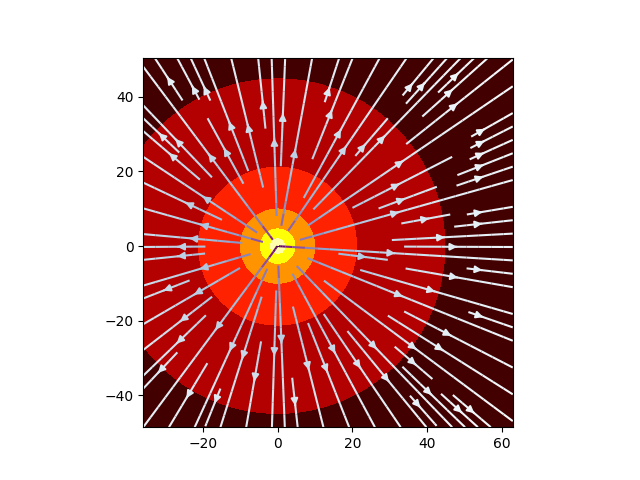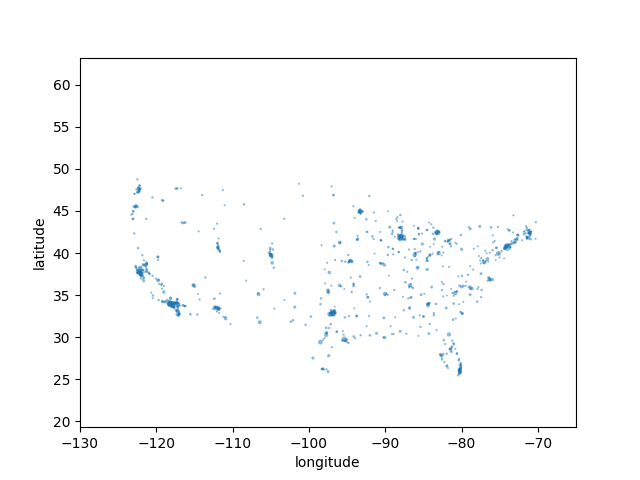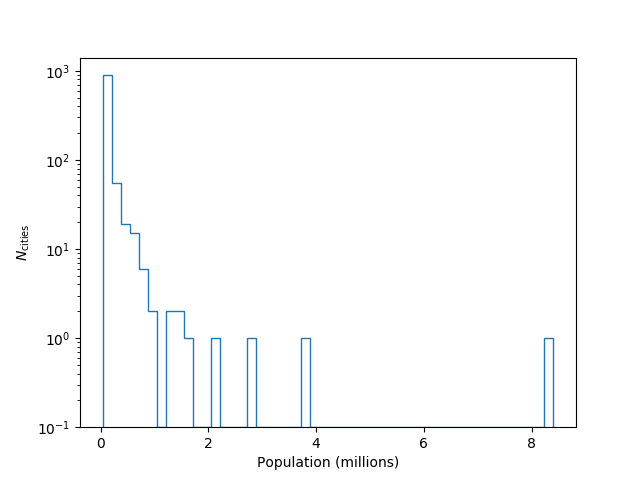This example demonstrates the basic usage of Trieste, with an emphasis on the added metadata that can be used for forensic analysis, or simply as a reminder to the author of the file.

In :
%pylab notebook

Populating the interactive namespace from numpy and matplotlib

In :
import trieste as tr


We'll begin by loading the Trieste file, demo.npz.

In :
demo = tr.load('demo.npz')

Warning: demo.npz was created with a newer version of Python.


Note the warning: The file was created with newer software than we are using, so it's possible that we could encounter a problem at some point. If we do encounter a problem, we can simply update our software and try again.

Let's see what is in the file by printing its table of contents (TOC) using print_toc().

In :
demo.print_toc()

 Index      Name               Type
-----------------------------------------
0 :    xvals              1-D Array
1 :    yvals              1-D Array
2 :    unit_vec           4-D Array
3 :    rate               3-D Array
4 :    city data          Table
-----------------------------------------


Let's see if the person who created this file included documentation, in the form of a README:

In :
print(demo.readme)

This file contains some data for demonstration purposes. For instance, it includes arrays of varying
dimensionality and a table. The arrays contain a 3-dimensional flow field. You can plot a cross section of the
data like this:

plt.contourf(xvals, yvals, rate[50,:,:], cmap='hot')
plt.streamplot(xvals, yvals, unit_vec[0,50,:,:], unit_vec[1,50,:,:], color=rate[50,:,:], cmap='BuPu')

The city_data table is unrelated to the other data.



The author did not include a huge amount of info in the README doc, but it is still somewhat helpful. Let's see what metadata keys are available...

In :
demo.metadata.keys()

Out:
dict_keys(['doc', 'numpy_version', 'python_version', 'n_objects', 'trieste_version', 'creation_time', 'creation_date', 'author', 'author_working_dir', 'hostname', 'OS', 'architecture', 'platform', 'python_implementation', 'ipython_history'])

It looks like we can find out who created this file:

In :
demo.metadata['author']

Out:
'nemo'

So, the user named 'Nemo' is the author. What machine were they using? ...

In :
demo.metadata['hostname']

Out:
'nautilus'

Of course. And what was the working directory? ...

In :
demo.metadata['author_working_dir']

Out:
'/home/nemo'

Nemo was working in his home directory on nautilus. What kind of machine is nautilus? ...

In :
demo.metadata['platform']

Out:
'Linux-4.14.14-1-x86_64-with-glibc2.3.4'

Linux 4.14 on an x86-64 CPU. When was the file created?

In :
demo.metadata['creation_date']

Out:
'Mon Mar  5 22:49:29 2018'

If Nemo was using IPython or a Jupyter notebook when he created this file, we can find out exactly how he created the file...

In :
demo.print_history()

import json
import numpy as np
import trieste as tr
import sim
city_data = open("cities.json")

city_data.close()

city_table = np.ndarray(shape=(len(cities),), dtype=[('city', '<U20'),
('longitude', np.float32),
('latitude', np.float32),
('population', int)])

for i, city in enumerate(cities):
city_table[i] = city['city'], city['longitude'], city['latitude'], city['population']

table = tr.Table(city_table, 'city data')

table.set_readme('This file contains the coordinates and populations of 1000 cities.')

xvals, yvals, unit_vec, rate = sim.flow_field((36, 48.5, 68.5))

x = tr.Array(xvals)

x.set_readme("The x-coordinates of the center of each voxel in the flow field grid.")

y = tr.Array(yvals)

y.set_readme("The y-coordinates of the center of each voxel in the flow field.")

unit_vec = tr.Array(unit_vec)

unit_vec.set_readme("Unit vectors, pointing in the direction of flow at each sample point within the volume.")

flow_rate = tr.Array(rate)

flow_rate.set_readme("The flow rate of the fluid at each sample point (voxel centers) within the volume.")

notes = """This file contains some data for demonstration purposes. For instance, it includes arrays of varying
dimensionality and a table. The arrays contain a 3-dimensional flow field. You can plot a cross section of the
data like this:

plt.contourf(xvals, yvals, rate[50,:,:], cmap='hot')
plt.streamplot(xvals, yvals, unit_vec[0,50,:,:], unit_vec[1,50,:,:], color=rate[50,:,:], cmap='BuPu')

The city_data table is unrelated to the other data.

"""

tr.save("demo", {'doc':notes}, x, y, unit_vec, flow_rate, table)


This gives us much more info than the README document! Nemo loaded a JSON file named cities.json and then parsed the file to create the table included in this Trieste file. The cities.json file was apparently located in his home directory.

In order to create the arrays in the file, Nemo loaded a Python module called sim and executed sim.flow_field((36, 48.5, 68.5)). So, this nautilus machine contains a Python module called sim which we can examine in further detail, if we can gain access to the machine.

Okay, let's look at the contents of the file again...

In :
demo.print_toc()

 Index      Name               Type
-----------------------------------------
0 :    xvals              1-D Array
1 :    yvals              1-D Array
2 :    unit_vec           4-D Array
3 :    rate               3-D Array
4 :    city data          Table
-----------------------------------------


In the README, Nemo suggested plotting the flow field, stored in the arrays. Let's try that. We can access the content of each array by indexing them with an integer, as in:

In :
xvals = demo

In :
xvals

Out:
<xvals: a Trieste 1-D Array>

To get the actual data stored in the array, we use the data attribute:

In :
xvals.data

Out:
array([-36., -35., -34., -33., -32., -31., -30., -29., -28., -27., -26.,
-25., -24., -23., -22., -21., -20., -19., -18., -17., -16., -15.,
-14., -13., -12., -11., -10.,  -9.,  -8.,  -7.,  -6.,  -5.,  -4.,
-3.,  -2.,  -1.,   0.,   1.,   2.,   3.,   4.,   5.,   6.,   7.,
8.,   9.,  10.,  11.,  12.,  13.,  14.,  15.,  16.,  17.,  18.,
19.,  20.,  21.,  22.,  23.,  24.,  25.,  26.,  27.,  28.,  29.,
30.,  31.,  32.,  33.,  34.,  35.,  36.,  37.,  38.,  39.,  40.,
41.,  42.,  43.,  44.,  45.,  46.,  47.,  48.,  49.,  50.,  51.,
52.,  53.,  54.,  55.,  56.,  57.,  58.,  59.,  60.,  61.,  62.,
63.])

So, let's load the data for all of the arrays. Note that we can also access the data in the file by specifying the name of the array:

In :
xvals = xvals.data
yvals = demo['yvals'].data

In :
unit_vec = demo.data
rate = demo['rate'].data


Now, let's plot the flow field, as Nemo suggested:

In :
plt.contourf(xvals, yvals, rate[50,:,:], cmap='hot')
plt.streamplot(xvals, yvals, unit_vec[0,50,:,:], unit_vec[1,50,:,:], color=rate[50,:,:], cmap='BuPu')
plt.axis('square')Out:
(-36.0, 63.0, -48.5, 50.5)

Nemo seems to be showing us the flow field from an explosion or something!

In :
plt.close()


Now let's look at the city data table:

In :
city_data = demo

In :
print(city_data.readme)

This file contains the coordinates and populations of 1000 cities.

In :
city_data

Out:
<city data: a Trieste Table>

What are the columns of the table? ...

In :
city_data.column_names

Out:
('city', 'longitude', 'latitude', 'population')

What are the data types of each column? ...

In :
city_data.column_types

Out:
{'city': dtype('<U20'),
'latitude': dtype('float32'),
'longitude': dtype('float32'),
'population': dtype('int64')}

Let's look at the first 10 entries in the table, to get a feel for what's inside...

In :
city_data[:10]

Out:
array([('New York',  -74.00594 , 40.712784, 8405837),
('Los Angeles', -118.24368 , 34.052235, 3884307),
('Chicago',  -87.6298  , 41.878113, 2718782),
('Houston',  -95.369804, 29.760427, 2195914),
('Phoenix', -112.074036, 33.448376, 1513367),
('San Antonio',  -98.49363 , 29.424122, 1409019),
('San Diego', -117.16109 , 32.715736, 1355896),
('Dallas',  -96.79699 , 32.776665, 1257676),
('San Jose', -121.88633 , 37.338207,  998537)],
dtype=[('city', '<U20'), ('longitude', '<f4'), ('latitude', '<f4'), ('population', '<i8')])

Oh! It looks like this is a list of US cities, sorted from highest population to lowest.

This table contains city coordinates, so we can create a scatter plot and scale the points so that cities with larger populations are larger...

In :
lon = city_data['longitude']
lat = city_data['latitude']
pop = city_data['population']

In :
sqrtpop = np.sqrt(pop)
normed_sqrtpop = sqrtpop/sqrtpop.max()
plt.scatter(lon, lat, s=30*normed_sqrtpop, linewidths=0, alpha=0.5)
plt.xlim((-130,-65))
plt.xlabel('longitude')
plt.ylabel('latitude')Out:
Text(0,0.5,'latitude')
In :
plt.close()


We can also look at the distribution of populations...

In :
plt.hist(pop/1e6, bins=50, histtype='step', log=True)
plt.xlabel("Population (millions)")
plt.ylabel(r"$N_{\rm cities}$")Out:
Text(0,0.5,'$N_{\\rm cities}$')
In :
plt.close()


New York City (the one on the far right) is quite an outlier!

We could have also obtained a NumPy RecArray, like this:

In :
cities = city_data.as_recarray()


Then, we can do things like search for all cities named Pasadena:

In :
cities[cities.city == 'Pasadena']

Out:
rec.array([('Pasadena',  -95.2091  , 29.691063, 152735),
dtype=[('city', '<U20'), ('longitude', '<f4'), ('latitude', '<f4'), ('population', '<i8')])

and obtain a record for the 718th most populous city:

In :
cities

Out:
('Harrisonburg', -78.86891, 38.44957, 51395)

also:

In :
hburg = cities

In :
hburg.city

Out:
'Harrisonburg'
In :
hburg.longitude, hburg.latitude

Out:
(-78.86891, 38.44957)

To do more complicated things with tables, it's helpful to use Pandas:

In :
import pandas as pd

In :
cities = pd.DataFrame(city_data.data)

In :
cities[:15]

Out:
city longitude latitude population
0 New York -74.005943 40.712784 8405837
1 Los Angeles -118.243683 34.052235 3884307
2 Chicago -87.629799 41.878113 2718782
3 Houston -95.369804 29.760427 2195914
5 Phoenix -112.074036 33.448376 1513367
6 San Antonio -98.493629 29.424122 1409019
7 San Diego -117.161087 32.715736 1355896
8 Dallas -96.796989 32.776665 1257676
9 San Jose -121.886330 37.338207 998537
10 Austin -97.743057 30.267153 885400
11 Indianapolis -86.158066 39.768402 843393
12 Jacksonville -81.655647 30.332184 842583
13 San Francisco -122.419418 37.774929 837442
14 Columbus -82.998795 39.961174 822553
In :
cities.loc[cities.city == 'Pasadena']

Out:
city longitude latitude population